# MA2051 - Ordinary Differential EquationsReview 1

1. Solve the following initial value problems.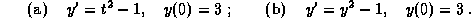2. The Worcester Yeast Corporation grows and sells a yeast used in beverage production. The yeast is grown in a very large vat, where it is provided with ample nutrient for growth. Assume that, in the absence of harvesting, the rate of growth of this yeast is proportional to its present population and that the yeast population will double its size in 4 hours.

(a)
Derive a model for the growth of the yeast population P (measured in kilograms) when the yeast is harvested at a constant rate H (kg/hour). (Note: Use the doubling time to determine the growth rate.)
(b)
If the harvest rate is too large, the yeast population will be used up in finite time (and you are out of business). Determine the largest constant harvest rate that will not use all of the yeast in a finite time. What is the maximum amount of yeast that you will harvest in an 8 hour day if the initial yeast population is 100 kg and you use a constant harvest rate?
(c)
Is it possible to harvest more yeast by using a nonconstant harvesting policy? Justify your answer.

3. Given thatsolves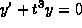with,
andsolves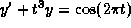with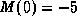,
find the solution, in terms of L and M, for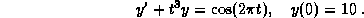4. Consider the following initial value problem: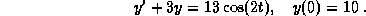(a)
Find a nontrivial homogeneous solution.
(b)
Use the method of undetermined coefficients to find a particular solution.
(c)
Find the solution to the initial value problem.
(d)
Describe the behavior of the solution for large values of the independent variable t. What is the transient component of the solution?

5. Consider the differential equation: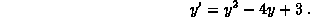(a)
Find all of the steady states for this equation.
(b)
Determine the regions in the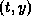-plane where the solution is increasing and where it is decreasing. Use this information to sketch a solution curve for each of three different initial points:,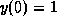, and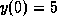.
(c)
Use your sketch to determine the stability of each steady state. Justify your answer.
(d)
Use one step of Euler's method to approximate the time required for the solution to decrease from an initial position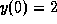to the level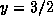.

Solutions To This Review
```

© 1996 by Will Brother.
All rights Reserved. File last modified on March 22, 1996.

```